You are currently offline. Some features of the site may not work correctly.

# Algebraic independence

Known as: Algebraically independent, Algebraically dependent, Algebraic dependence
In abstract algebra, a subset S of a field L is algebraically independent over a subfield K if the elements of S do not satisfy any non-trivial… Expand
Wikipedia

## Papers overview

Semantic Scholar uses AI to extract papers important to this topic.
2008
2008
Usually creative telescoping is used to derive recurrences for sums. In this article we show that the non-existence of a creative… Expand
Is this relevant?
Highly Cited
2005
Highly Cited
2005
Non-commutative polynomial algebras appear in a wide range of applications, from quantum groups and theoretical physics to linear… Expand
Is this relevant?
Highly Cited
2004
Highly Cited
2004
• 2004
• Corpus ID: 26328516
Let A = F [x1, x2, . . . , xn] be a ring of polynomials over a field F on the variables x1, x2, . . . , xn. It is well known (see… Expand
Is this relevant?
2002
2002
• 2002
• Corpus ID: 13200676
A general class of growing networks is constructed with both preferential and random attachments, which includes random and scale… Expand
•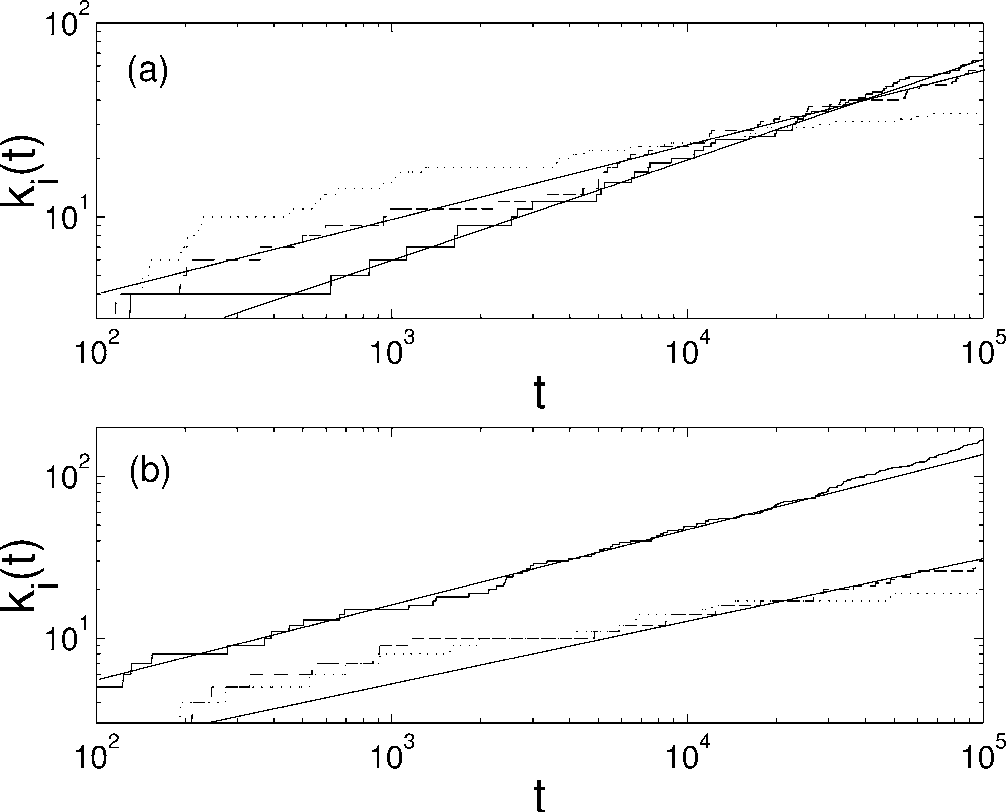•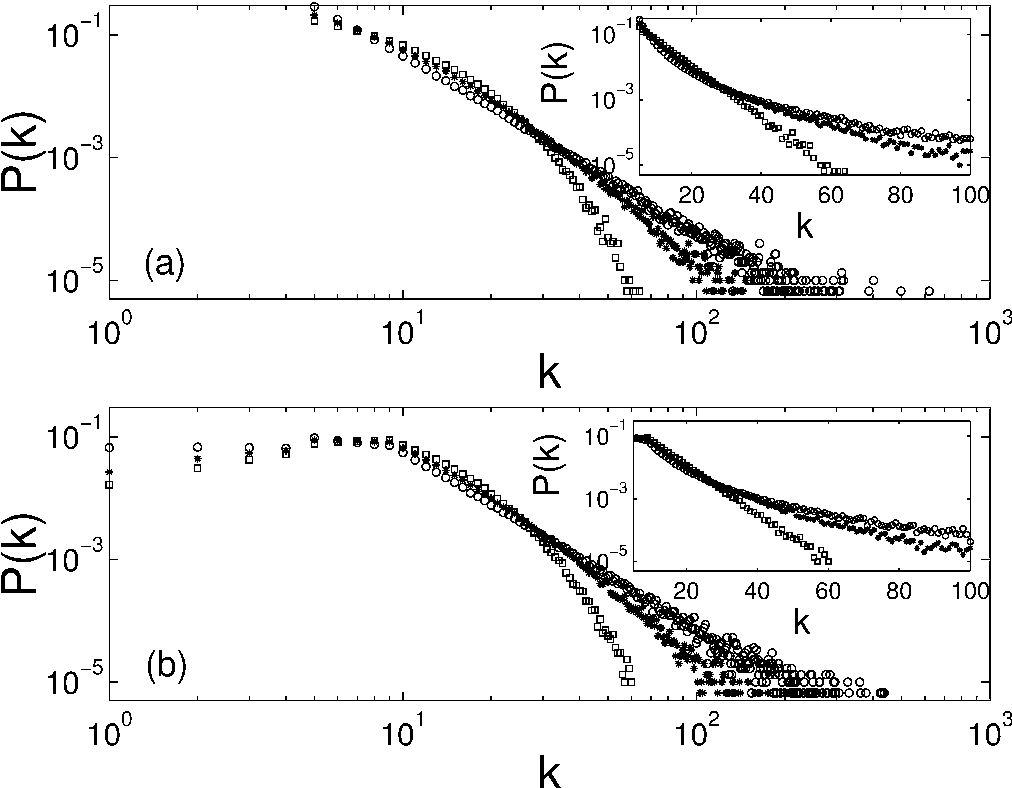•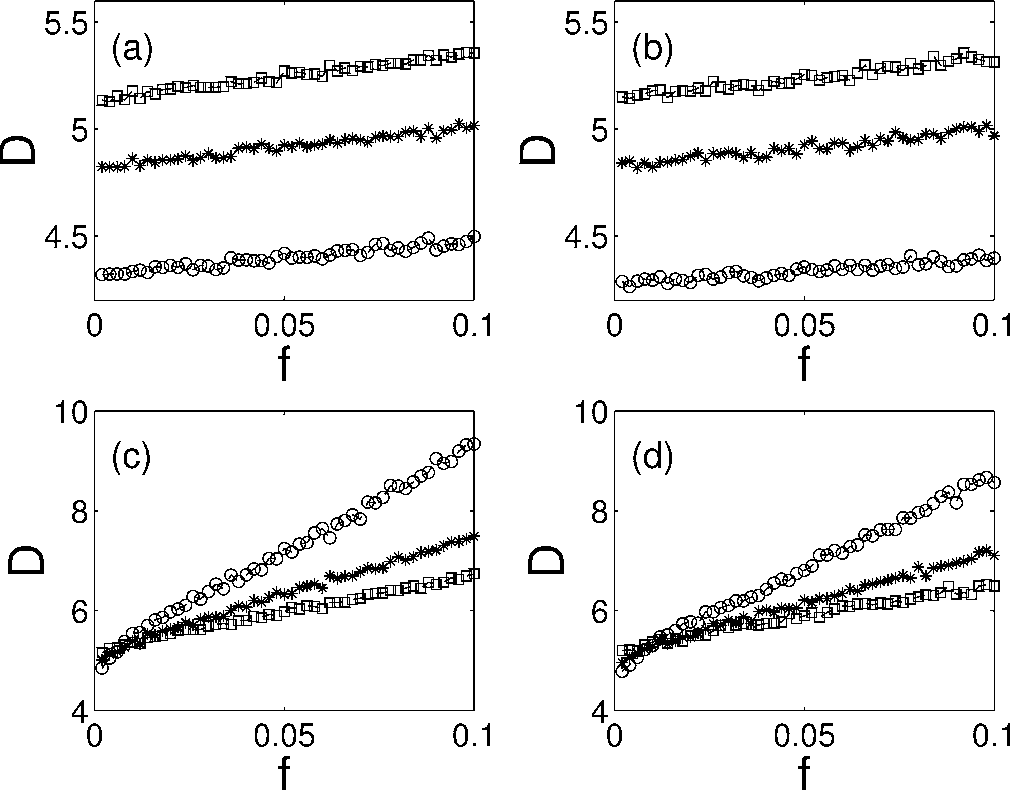Is this relevant?
Highly Cited
1999
Highly Cited
1999
• EUROCRYPT
• 1999
• Corpus ID: 206633235
We show that if the private exponent d used in the RSA public-key cryptosystem is less than N0:292 then the system is insecure… Expand
•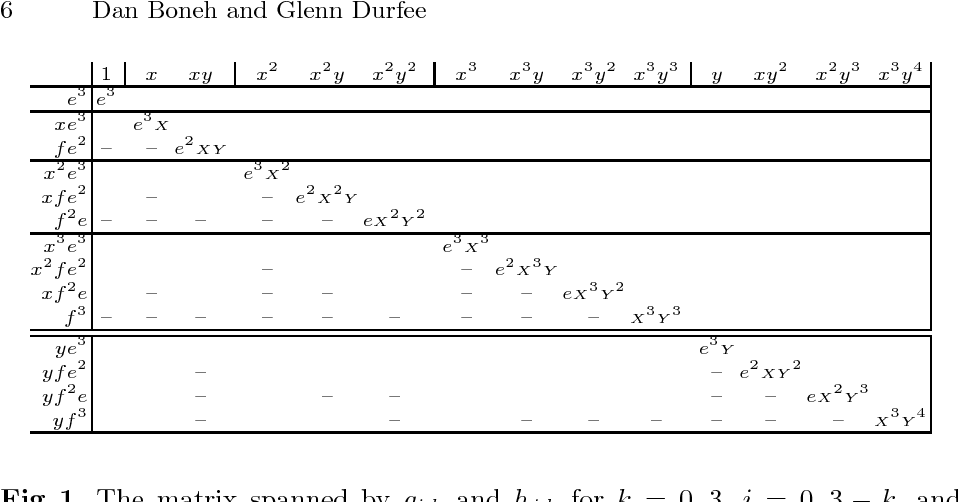Is this relevant?
1999
1999
• Jacques Stern
• Lecture Notes in Computer Science
• 1999
• Corpus ID: 29690672
We show that if the private exponent d used in the RSA public-key cryptosystem is less than N then the system is insecure. This… Expand
•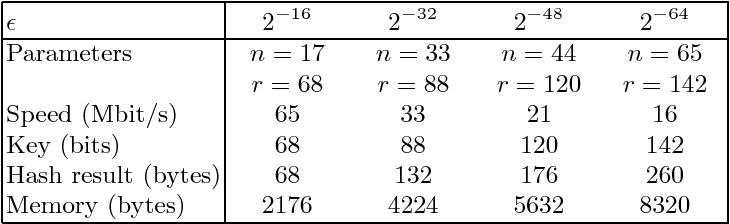•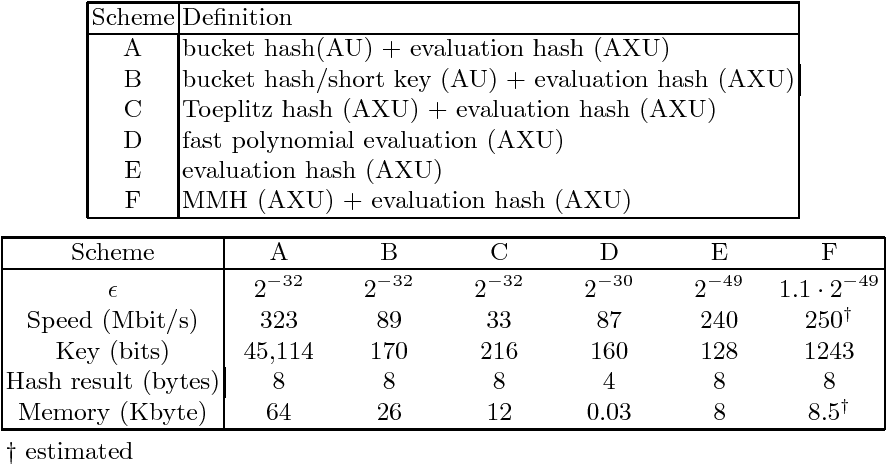•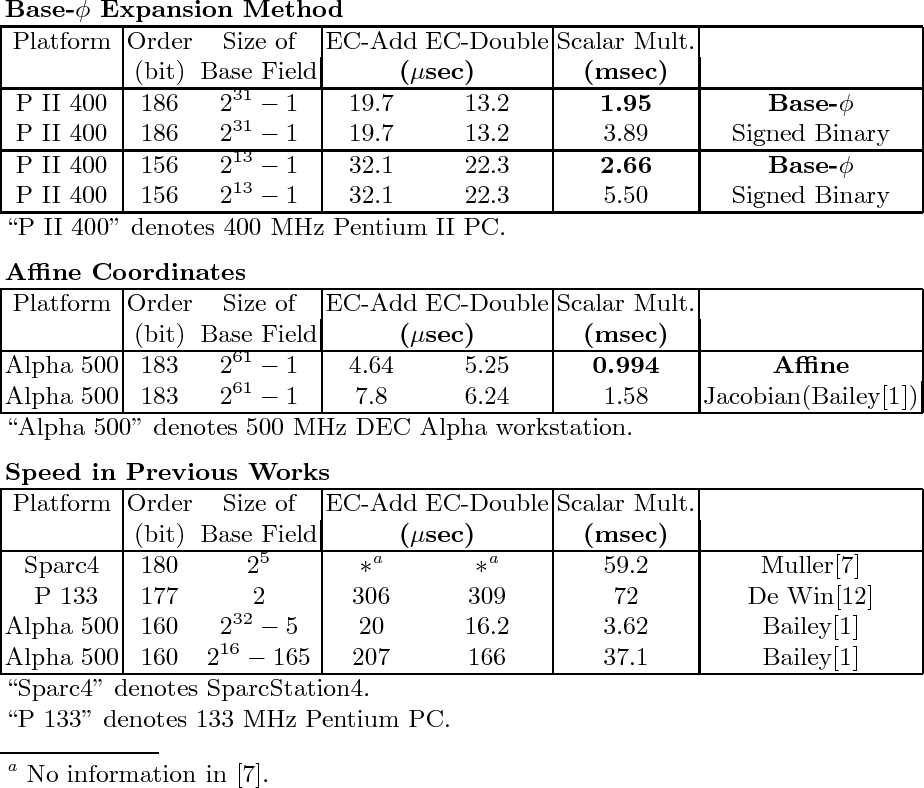•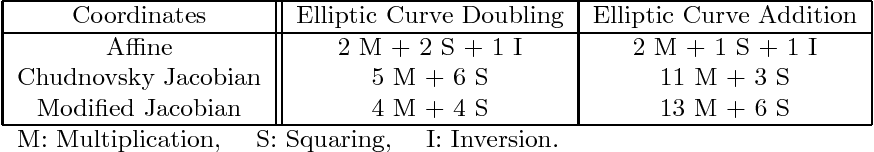•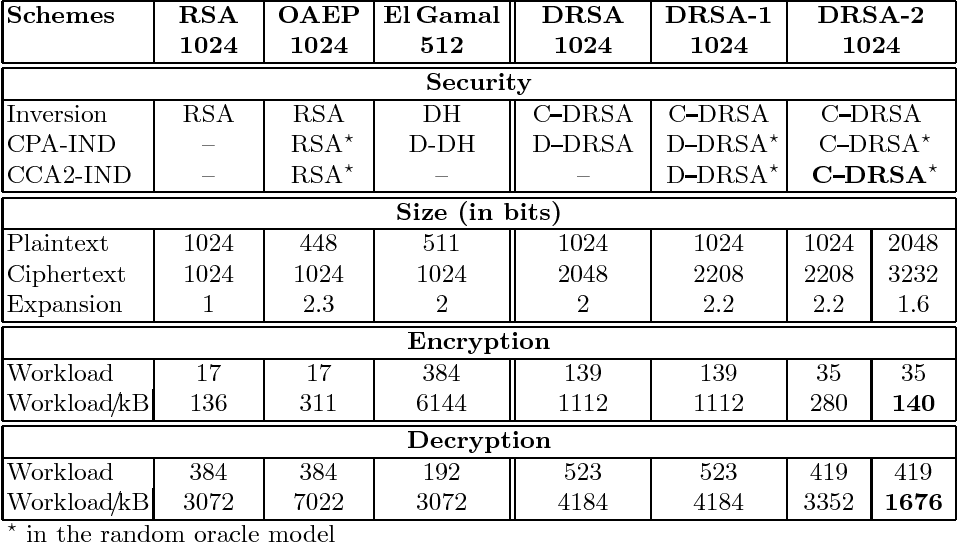Is this relevant?
1994
1994
Abstract Mutual stochastic independences among σ-algebras and mutual algebraic independences among elements of semimodular… Expand
Is this relevant?
1986
1986
This action of G defines an action of G on an algebra C[W] = C[xo(l), • , Xij(l)] of all polynomial functions on W. We denote by… Expand
Is this relevant?
Highly Cited
1974
Highly Cited
1974
Let the region S={(x, y)∥μ(x+iy, x−iy)>0} be the interior of Steiner's hypocycloid, where μ(z, z)=−z2z2+4z3+4z3−18zz+27. For each… Expand
•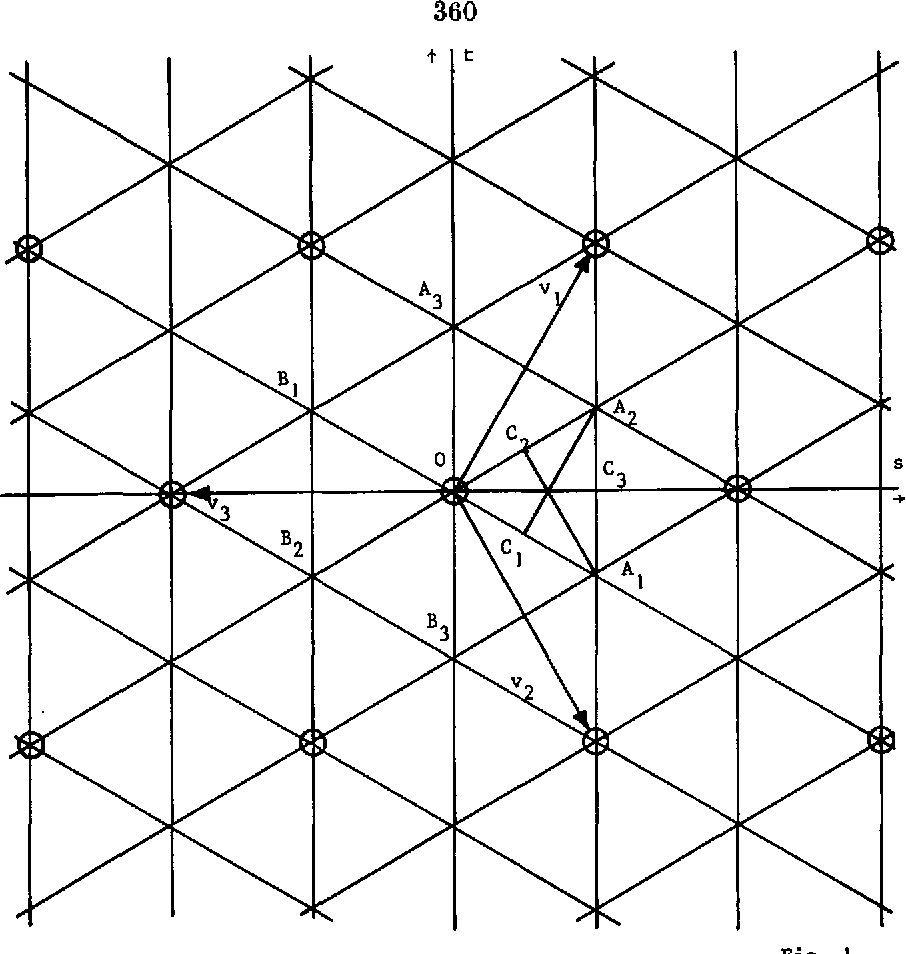Is this relevant?
Highly Cited
1964
Highly Cited
1964
PROOF. Approximate fi, * *, fm by real polynomials F1, * , Fm of the same degrees whose coefficients are algebraically… Expand
Is this relevant?